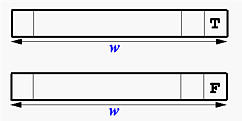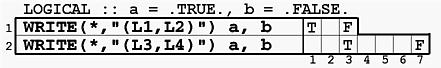# LOGICAL Output: The L DescriptorThe Lw descriptor is for LOGICAL output. While Fortran uses .TRUE. and .FALSE. to indicate logical values true and false, respectively, the output only show T and F. The general form of this descriptor is as follows:

rLw

The meaning of r and w are:

• L is for LOGICAL
• w is the width of field, which indicates that a logical value should be printed with w positions.
• The output of a LOGICAL value is either T for .TRUE. or F for .FALSE. The single character value is shown in the right-most position and the remaining w-1 positions are filled with spaces. The is shown in the figure below.• r is the repetition indicator, which gives the number of times the edit descriptor should be repeated. For example, 3I5.3 is equivalent to I5.3, I5.3, I5.3.### Examples

Let us look at the following example. There are two LOGICAL variables a and b with values .TRUE. and .FALSE., respectively. In the following table, the WRITE statements are shown in the left and their corresponding output are shown in the right.• The first WRITE uses L1 and L2 to print .TRUE. and .FALSE., respectively. Therefore, a T is shown in position one (L1) and a F is shown in position three (L2).
• The second WRITE uses L3 and L4 to print .TRUE. and .FALSE.. Therefore, a T is printed in the third position of the first three positions, and a F is printed in the fourth position of the next four positions. There are seven positions used in total.